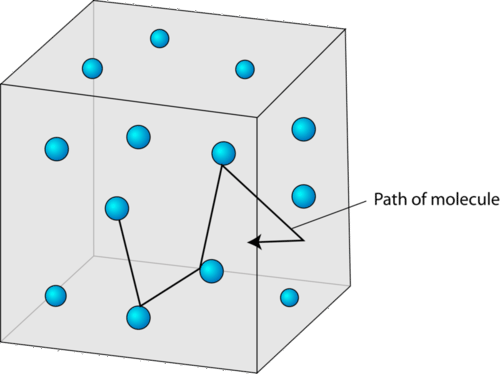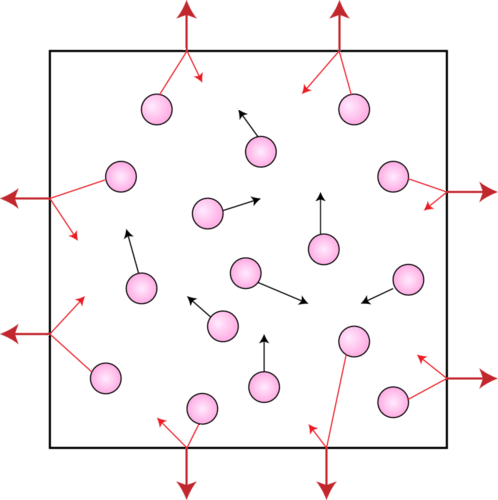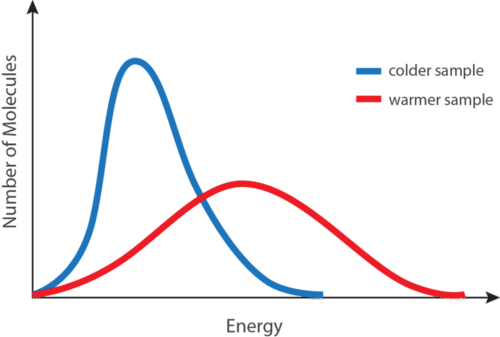# 7.3: Kinetic-Molecular Theory

•• Contributed by Allison Soult
• Senior Lecturer (Chemistry) at University of Kentucky

Learning Outcomes

• Define pressure and describe how gases exert pressure.
• Convert between units of gas pressure ($$\text{atm}$$, $$\text{mm} \ce{Hg}$$, $$\text{torr}$$, and $$\text{Pa}$$).
• Relate temperature to average kinetic energy.
• Relate mass to molecular speed.
• Describe the behavior of an ideal gas.

The kinetic-molecular theory is a theory that explains the states of matter and is based on the idea that matter is composed of tiny particles that are always in motion. The theory helps explain observable properties and behaviors of solids, liquids, and gases. However, the theory is most easily understood as it applies to gases. The theory applies specifically to a model of a gas called an ideal gas. An ideal gas is an imaginary gas whose behavior perfectly fits all the assumptions of the kinetic-molecular theory. In reality, gases are not ideal, but they are very close to being so under most everyday conditions.

The kinetic-molecular theory, as it applies to gases, has five basic assumptions.

1. Gases consist of very large numbers of tiny spherical particles that are far apart from one another compared to their size. The particles of a gas may be either atoms or molecules. The distance between the particles of a gas is much, much greater than the distances between the particles of a liquid or a solid. Most of the volume of a gas, therefore, is composed of the empty space between the particles. In fact, the volume of the particles themselves is considered to be insignificant compared to the volume of the empty space.
2. Gas particles are in constant rapid motion in random directions. The fast motion of gas particles gives them a relatively large amount of kinetic energy. Recall that kinetic energy is the energy that an object possesses because of its motion. The particles of a gas move in a straight line until they collide with another particle or with one of the walls of their container (see figure below).
3. Collisions between gas particles and between particles and the container walls are elastic collisions. An elastic collision is one in which there is no overall loss of kinetic energy. Kinetic energy may be transferred from one particle to another during an elastic collision, but there is no change in the total energy of the colliding particles.
4. There are no forces of attraction or repulsion between gas particles. Attractive forces are responsible for particles of a real gas condensing together to form a liquid. It is assumed that the particles of an ideal gas have no such attractive forces. The motion of each particle is completely independent of the motion of all other particles.
5. The average kinetic energy of gas particles is dependent upon the temperature of the gas. As the temperature of a gas is increased, its component particles begin to move faster, resulting in an increase in their kinetic energies. Not all particles in a given sample have the same speed, so the sample will contain particles with a range of different kinetic energies. However, the average kinetic energy of the particles in a sample is proportional to its temperature.Figure $$\PageIndex{1}$$: Gas particles move in a random, linear fashion according to the kinetic-molecular theory. The space between particles is very large compared to the size of the particles.

## Gas Pressure

Pressure is defined as the force per unit area on a surface.

$\text{Pressure} = \frac{\text{force}}{\text{area}}$

When a person stands on the floor, his feet exert pressure on the surface. That pressure is related to both the mass of the person and the surface area of his feet. If the person were holding a heavy object, the pressure would increase because of a greater force. Alternatively, if the person stands on his toes, the pressure also increases because of a decrease in the surface area.

Gas molecules also exert pressure. Earth's atmosphere exerts pressure because gravity acts on the huge number of gas particles contained in the atmosphere, holding it in place. Pressure is also exerted by small samples of gas, such as the outward pressure exerted by the gas inside a balloon. Gas pressure is the pressure that results from collisions of gas particles with an object. Inside the balloon, the gas particles collide with the balloon's inner walls. It is those collisions that keep the balloon inflated. If the gas particles were to suddenly stop moving, the balloon would instantly deflate. The figure below is an illustration of gas particles exerting pressure inside a container.Figure $$\PageIndex{2}$$: Gas pressure results from collisions with the surface of its container.

### Measuring Pressure

Atmospheric pressure is the pressure exerted by the gas particles in Earth's atmosphere as those particles collide with objects. A barometer is an instrument used to measure atmospheric pressure. A traditional mercury barometer consists of an evacuated tube immersed in a container of mercury. Air molecules from the atmosphere push down on the outer surface of the mercury, but, because the inside of the tube is a vacuum, there is no corresponding downward push on the mercury in the tube. As a result, the mercury rises inside the tube. The height to which the mercury rises is dependent on the external air pressure.

At sea level, a mercury column will rise a distance of $$760 \: \text{mm}$$. This atmospheric pressure is reported as $$760 \: \text{mm} \: \ce{Hg}$$ (millimeters of mercury). At higher altitudes, the atmospheric pressure is lower, so the column of mercury will not rise as high. For example, on the summit of Mt. Everest (at an elevation of $$8848 \: \text{m}$$), the air pressure is $$253 \: \text{mm} \: \ce{Hg}$$. Atmospheric pressure is also slightly dependent on weather conditions.

A more convenient barometer, called an aneroid barometer, measures pressure by the expansion and contraction of a small spring within an evacuated metal capsule.

### Units of Gas Pressure

A barometers measures gas pressure by the height of the column of mercury. One unit of gas pressure is the millimeter of mercury $$\left( \text{mm} \: \ce{Hg} \right)$$. An equivalent unit to the $$\text{mm} \: \ce{Hg}$$ is called the torr, in honor of the inventor of the barometer, Evangelista Torricelli. The pascal $$\left( \text{Pa} \right)$$ is the standard unit of pressure. A pascal is a very small amount of pressure, so a more useful unit for everyday gas pressures is the kilopascal $$\left( \text{kPa} \right)$$. A kilopascal is equal to 1000 pascals. Another commonly used unit of pressure is the atmosphere $$\left( \text{atm} \right)$$. Standard atmospheric pressure is called $$1 \: \text{atm}$$ of pressure and is equal to $$760 \: \text{mm} \: \ce{Hg}$$ and $$101.3 \: \text{kPa}$$. The relationships between the most common units of pressure are shown below.

$1 \: \text{atm} = 760 \: \text{mm} \: \ce{Hg} = 760 \: \text{torr} = 101.3 \: \text{kPa}$

Example $$\PageIndex{1}$$

The atmospheric pressure in a mountainous location is measured to be $$613 \: \text{mm} \: \ce{Hg}$$. What is this pressure in $$\text{atm}$$ and in $$\text{kPa}$$?

Solution

Step 1: List the known quantities and plan the problem.

Known

• Given: $$613 \: \text{mm} \: \ce{Hg}$$
• $$1 \: \text{atm} = 760 \: \text{mm} \: \ce{Hg}$$
• $$101.3 \: \text{kPa} = 760 \: \text{mm} \: \ce{Hg}$$

Unknown

• Pressure $$= ? \: \text{atm}$$
• Pressure $$= ? \: \text{kPa}$$

Use conversion factors from the equivalent pressure units to convert from $$\text{mm} \: \ce{Hg}$$ to $$\text{atm}$$ and from $$\text{mm} \: \ce{Hg}$$ to $$\text{kPa}$$.

Step 2: Solve.

$613 \: \text{mm} \: \ce{Hg} \times \frac{1 \: \text{atm}}{760 \: \text{mm} \: \ce{Hg}} = 0.807 \: \text{atm} \nonumber$

$613 \: \text{mm} \: \ce{Hg} \times \frac{101.3 \: \text{kPa}}{760 \: \text{mm} \: \ce{Hg}} = 81.7 \: \text{kPa} \nonumber$

The air pressure is about $$80\%$$ of the standard atmospheric pressure at sea level. The standard pressure of $$760 \: \text{mm} \: \ce{Hg}$$ can be considered to have three significant figures.

## Kinetic Energy and Temperature

As stated in the kinetic-molecular theory, the temperature of a substance is related to the average kinetic energy of the particles of that substance. When a substance is heated, some of the absorbed energy is stored within the particles, while some of the energy increases the speeds at which the particles are moving. This is observed as an increase in the temperature of the substance.

### Average Kinetic Energy

At any given temperature, not all of the particles in a sample of matter have the same kinetic energy. Instead, the particles display a wide range of kinetic energies. Most of the particles have a kinetic energy near the middle of the range. However, some of the particles have kinetic energies a great deal lower or a great deal higher than the average (see figure below).Figure $$\PageIndex{3}$$: A distribution of molecular kinetic energies as a function of temperature. The blue curve is for a low temperature, while the red curve is for a high temperature.

The blue curve shown (see figure above) is for a sample of matter at a relatively low temperature, while the red curve is for a sample at a relatively high temperature. In both cases, most of the particles have intermediate kinetic energies, close to the average. Notice that as temperature increases, the range of kinetic energies increases and the distribution curve "flattens out".

At a given temperature, the particles of any substance have the same average kinetic energy. At room temperature, the molecules in a sample of liquid water have the same average kinetic energy as the molecules in a sample of oxygen gas or the ions in a sample of sodium chloride.

### Absolute Zero

As a sample of matter is continually cooled, the average kinetic energy of its particles decreases. Eventually, one would expect the particles to stop moving completely. Absolute zero is the temperature at which the motion of particles theoretically ceases. Absolute zero has never been attained in the laboratory, but temperatures on the order of $$1 \times 10^{-10} \: \text{K}$$ have been achieved. The Kelvin temperature scale is based on this theoretical limit, so absolute zero is equal to $$0 \: \text{K}$$. The Kelvin temperature of a substance is directly proportional to the average kinetic energy of the particles of the substance. For example, the particles in a sample of hydrogen gas at $$200 \: \text{K}$$ have twice the average kinetic energy as the particles in a hydrogen sample at $$100 \: \text{K}$$.